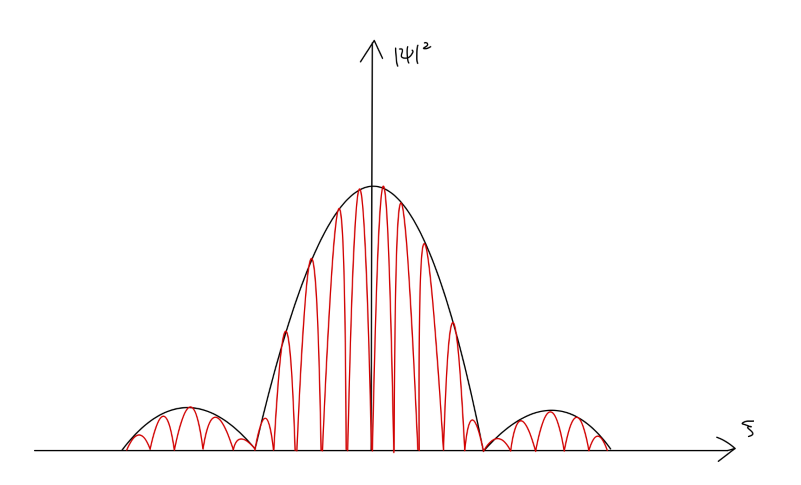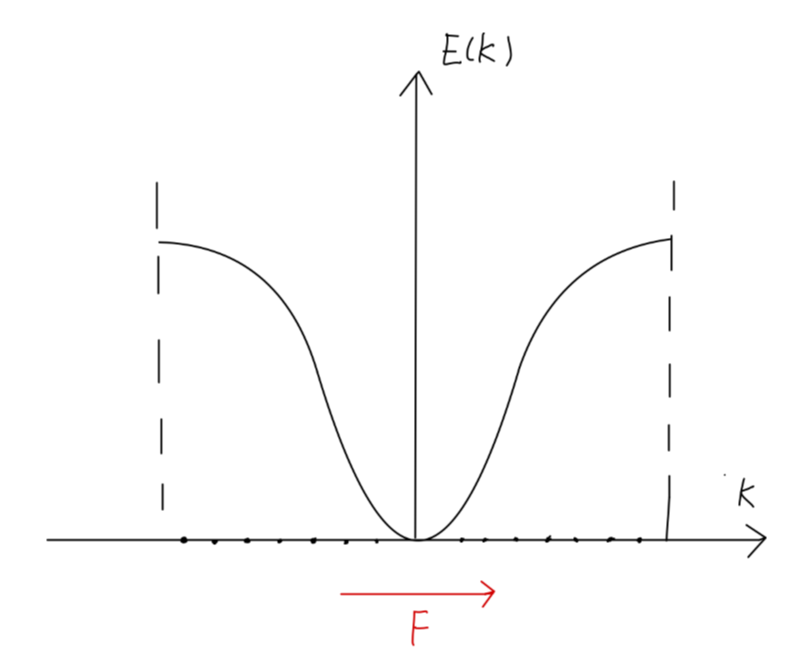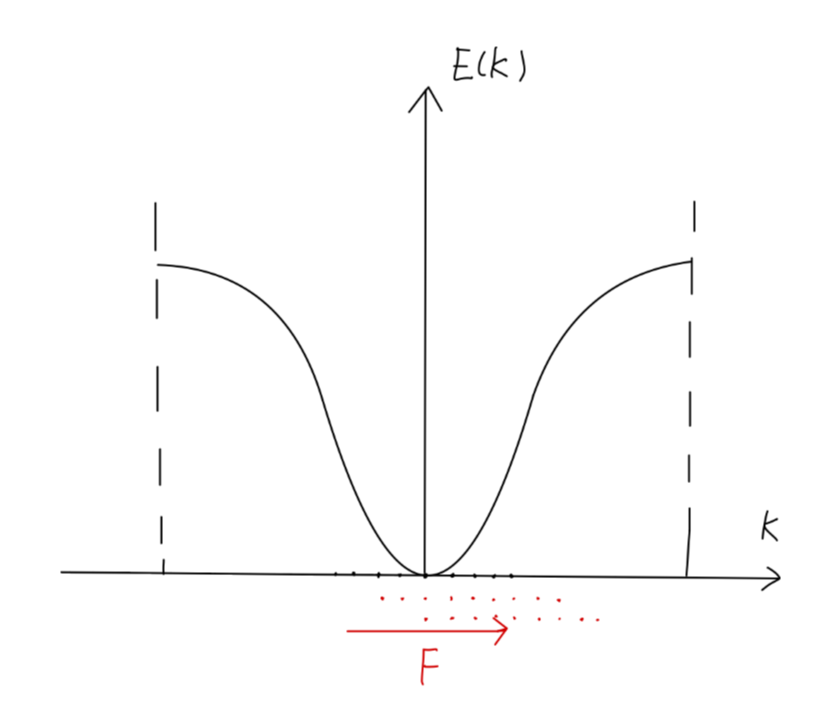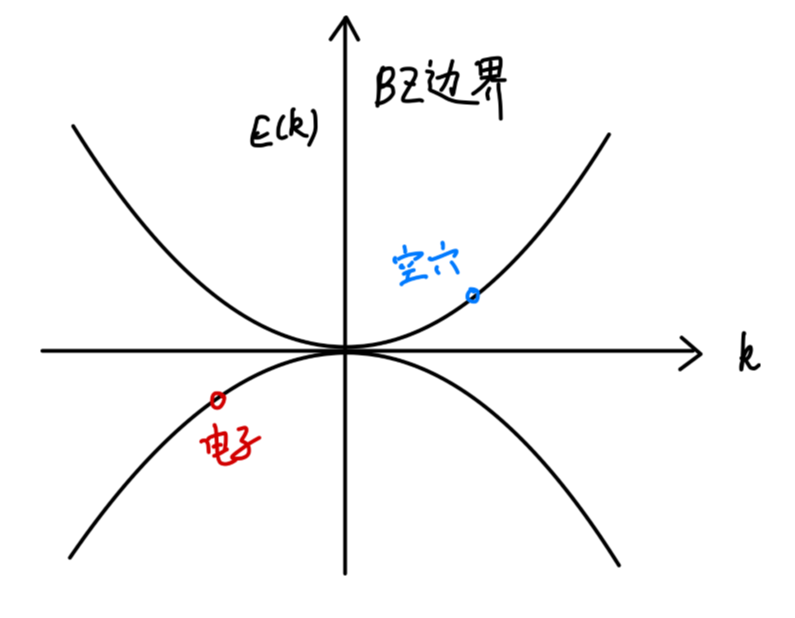## Bloch 电子的动力学性质

### 准经典近似

$\psi_{\bm{k}n}(\bm{r},t) = e^{i[\bm{k}\cdot \bm{r} - \frac{E_n(\bm{k})}{\hbar}t]}u_{\bm{k}n}(\bm{r}) \tag{1}$

\begin{aligned} \psi_{\bm{k}n}(\bm{r},t) &= \frac{1}{\Delta \bm{k}} \int_{\bm{k}_0 - \frac{\Delta \bm{k}}{2}}^{\bm{k}_0 + \frac{\Delta \bm{k}}{2}}e^{i[\bm{k}\cdot \bm{r} - \frac{E_n(\bm{k})}{\hbar}t]}u_{\bm{k}n}(\bm{r}) d\bm{k}\\ & \approx \frac{u_{\bm{k}n}(\bm{r})}{\Delta \bm{k}}e^{i[\bm{k}_0\cdot \bm{r} - \frac{E_n(\bm{k}_0)}{\hbar}t]} \int_{- \frac{\Delta \bm{k}}{2}}^{\frac{\Delta \bm{k}}{2}} e^{i[\delta\bm{k}\cdot (\bm{r} - \frac{[\nabla_{\bm{k}}E_n(\bm{k})]_{\bm{k}_0}}{\hbar}t)]} d\delta\bm{k}\\ \end{aligned}\tag{2}

\left\{ \begin{aligned} \xi & = x - \frac{1}{\hbar}(\frac{\partial E_n(\bm{k})}{\partial k_x})_{\bm{k}_0} t\\ \eta & = y - \frac{1}{\hbar}(\frac{\partial E_n(\bm{k})}{\partial k_y})_{\bm{k}_0} t\\ \zeta & = z - \frac{1}{\hbar}(\frac{\partial E_n(\bm{k})}{\partial k_z})_{\bm{k}_0} t\\ \end{aligned} \right.\tag{3}

\begin{aligned} \psi_{\bm{k}n}(\bm{r},t) &\approx \psi_{\bm{k}_0n}(\bm{r},t) \frac{\sin (\frac{\Delta k_x}{2} \xi)}{\frac{\Delta k_x}{2} \xi}\frac{\sin (\frac{\Delta k_y}{2} \eta)}{\frac{\Delta k_y}{2} \eta}\frac{\sin (\frac{\Delta k_z}{2} \zeta)}{\frac{\Delta k_z}{2} \zeta}\\ & = \psi_{\bm{k}_0n}(\bm{r},t) A(\bm{r},t)\\ \end{aligned}\tag{4}Fig：布洛赫波包

\left\{ \begin{aligned} x &= \frac{1}{\hbar}(\frac{\partial E_n(\bm{k})}{\partial k_x})_{\bm{k}_0} t\\ y &= \frac{1}{\hbar}(\frac{\partial E_n(\bm{k})}{\partial k_y})_{\bm{k}_0} t\\ z &= \frac{1}{\hbar}(\frac{\partial E_n(\bm{k})}{\partial k_z})_{\bm{k}_0} t\\ \end{aligned} \right.\tag{5}

$\bm{r} = \frac{1}{\hbar} \nabla E_n(\bm{k})_{\bm{k}_0}t\tag{6}$

$\bm{v} = \dot{\bm{r}} = \frac{1}{\hbar} \nabla E_n(\bm{k})_{\bm{k}_0}\tag{7}$

• 外场是时间和空间的缓变函数，外场变化的波长 $\lambda \gg a$
• 频率 $\hbar\omega \ll E_g$，以禁止带间跃迁。

### 在外力作用下 Bloch 电子状态的变化和准动量

$\bm{F}\cdot d\bm{s} = \bm{F}\cdot\bm{v}_{\bm{k}}dt$

$d E(\bm{k}) = \nabla E(\bm{k}) \cdot d\bm{k}$

$\nabla E(\bm{k}) \cdot d\bm{k} = \bm{F}\cdot\bm{v}_{\bm{k}}dt$

$\bm{v}_{\bm{k}} = \frac{1}{\hbar}\nabla_{\bm{k}}E(\bm{k})$

$(\hbar \frac{d\bm{k}}{dt} - \bm{F})\cdot \bm{v}_{\bm{k}} = 0$

$\frac{d}{dt}(\hbar\bm{k}) - \bm{F} = 0 \tag{8}$

### 加速度与有效质量

\begin{aligned} \frac{d\bm{v}}{dt} &= \frac{1}{\hbar} \frac{d}{dt}[\nabla_{\bm{k}}E(\bm{k})]\\ &= \frac{1}{\hbar}(\frac{d\bm{k}}{dt}\cdot\nabla_{\bm{k}})\nabla_{\bm{k}}E(\bm{k})\\ &= \frac{1}{\hbar^2}(\bm{F}\cdot\nabla_{\bm{k}})\nabla_{\bm{k}}E(\bm{k})\\ \end{aligned}\tag{9}

$\frac{dv_{\alpha}}{dt} = \sum_{\beta}\frac{1}{\hbar^2} \frac{\partial^2 E}{\partial k_{\alpha}\partial k_{\beta}} F_{\beta}\tag{10}$

$\begin{pmatrix} \dot{v}_x\\ \dot{v}_y\\ \dot{v}_z\\ \end{pmatrix} = \begin{pmatrix} \frac{1}{\hbar^2}\frac{\partial^2 E}{\partial k_{\alpha}\partial k_{\beta}} \end{pmatrix}_{3\times 3} \begin{pmatrix} F_x\\ F_y\\ F_z\\ \end{pmatrix}\tag{11}$

$\begin{pmatrix} F_x\\ F_y\\ F_z\\ \end{pmatrix} = \begin{pmatrix} \frac{1}{\hbar^2}\frac{\partial^2 E}{\partial k_{\alpha}\partial k_{\beta}} \end{pmatrix}_{3\times 3}^{-1} \begin{pmatrix} \dot{v}_x\\ \dot{v}_y\\ \dot{v}_z\\ \end{pmatrix}= \begin{pmatrix} m^*_{\alpha\beta} \end{pmatrix}_{3\times 3} \begin{pmatrix} \dot{v}_x\\ \dot{v}_y\\ \dot{v}_z\\ \end{pmatrix}\tag{12}$

$\{m_{\alpha\beta}^*\}$ 称为 有效质量张量

$(m^*_{\alpha\beta})^{-1} = \frac{1}{\hbar^2}\begin{pmatrix} \frac{\partial^2 E}{\partial k_x^2} & 0 & 0\\ 0 & \frac{\partial^2 E}{\partial k_y^2} & 0\\ 0 & 0 & \frac{\partial^2 E}{\partial k_z^2}\\ \end{pmatrix}$

$E(\bm{k}) = E_s - J_0 - 2J_1(\cos k_xa + \cos k_ya + \cos k_za)$

$(m^*_{\alpha\beta})^{-1} = \frac{1}{\hbar^2}\begin{pmatrix} 2a^2J_1 & 0 & 0\\ 0 & 2a^2J_1 & 0\\ 0 & 0 & 2a^2J_1\\ \end{pmatrix}$

$(m^*_{\alpha\beta})^{-1} = \frac{1}{\hbar^2}\begin{pmatrix} -2a^2J_1 & 0 & 0\\ 0 & -2a^2J_1 & 0\\ 0 & 0 & -2a^2J_1\\ \end{pmatrix}$

$(m^*_{\alpha\beta})^{-1} = \frac{1}{\hbar^2}\begin{pmatrix} 2a^2J_1 & 0 & 0\\ 0 & 2a^2J_1 & 0\\ 0 & 0 & -2a^2J_1\\ \end{pmatrix}$

## 导体、绝缘体和半导体的能带

### 满带电子不导电

$E(\bm{k}) = E(-\bm{k})$

$\bm{v}(\bm{k}) = -\bm{v}(-\bm{k})$

$I = \sum_{\bm{k}} -e\bm{v}(\bm{k}) = 0$Fig：满带中的电子运动

$\bm{F} = q\bm{E}$

$\frac{d\bm{k}}{dt} = \frac{\bm{F}}{\hbar}$

### 未满带电子导电Fig：未满带中的电子运动

### 导体、绝缘体、半导体的能带特征

• 导体：存在未满带
• 绝缘体：只有满带或空带，禁带宽 $5\sim7 eV$
• 半导体：只有满带或空带，禁带窄 $\sim 1eV$
• 半金属：导带低和价带顶有交叠

1. 每个初基胞中有奇数个价电子的固体：金属

2. 每个初基胞中有偶数个价电子的固体：

• 若有能带交叠：半金属
• 若无能带交叠：
• 宽禁带：绝缘体
• 窄禁带：半导体

#### 近满带和空穴

$\sum \bm{k} = 0$Fig：电子与空穴的能带

$\bm{k}_h= -\bm{k}_e \tag{13}$

$E_h(\bm{k}_h) = -E_e(\bm{k}_e) \tag{14}$

$\bm{v}_h(\bm{k}_{h}) = \bm{v}_e(\bm{k}_{e}) \tag{15}$

$m^*_{e} = -m^*_{h} \tag{16}$

## 其他计算能带的方法

### 平面波法

$\psi_{\bm{k}n} =e^{i\bm{k}\cdot\bm{r}} u_{\bm{k}n},\quad \bm{k}\in 1BZ$

Bloch 波为正空间的周期函数。

$u_{\bm{k}n}(\bm{r}) = u_{\bm{k}n}(\bm{r}+\bm{R}_l),\quad \bm{R}_l = \sum_i l_i \bm{a}_i\tag{17}$

$u_{\bm{k}n}(\bm{r}) = \frac{1}{\sqrt{N\Omega}}\sum_{\bm{K}_h} a_{\bm{k}}(\bm{K}_h) e^{i\bm{K}_h\cdot\bm{r}}\tag{18}$

$\psi_{\bm{k}n}(\bm{r}) = \frac{1}{\sqrt{N\Omega}}\sum_{\bm{K}_h} a_{\bm{k}}(\bm{K}_h) e^{i(\bm{k}+\bm{K}_h)\cdot\bm{r}}\tag{19}$

\begin{aligned} a_{\bm{k}}(\bm{K}_h) &= \frac{1}{\sqrt{N\Omega}}\int u_{\bm{k}n}(\bm{r})e^{-i\bm{K}_h\cdot\bm{r}}d\bm{r}\\ &= \frac{1}{\sqrt{N\Omega}} \int \psi_{\bm{k}n}(\bm{r})e^{i(\bm{k}+\bm{K}_h)\cdot\bm{r}} d\bm{r}\\ &= \frac{1}{\sqrt{N\Omega}} \int \psi_{\bm{k}+\bm{K}_h,n}(\bm{r})e^{i(\bm{k}+\bm{K}_h)\cdot\bm{r}} d\bm{r}\\ &= a_{\bm{k}}(\bm{k}+\bm{K}_h) \end{aligned}

$\psi_{\bm{k}n}(\bm{r}) = \frac{1}{\sqrt{N\Omega}}\sum_{\bm{K}_h} a_{\bm{k}}(\bm{k}+\bm{K}_h) e^{i(\bm{k}+\bm{K}_h)\cdot\bm{r}}$

$|\psi_{\bm{k}}\rangle = \sum_{\bm{K}_h}a(\bm{k}+\bm{K}_h) |\bm{k}+\bm{K}_h\rangle \tag{20}$

$\hat{H} = -\frac{\hbar^2}{2m}\nabla^2 + V(\bm{r}) = \hat{T} + V$

$\sum_{\bm{K}_h} a(\bm{k}+\bm{K}_n)(\hat{T} + V - E)|\bm{k}+\bm{K}_n\rangle = 0 \tag{21}$

\left\{ \begin{aligned} &\langle \bm{k} + \bm{K}_m | \bm{k} + \bm{K}_n \rangle = \delta_{\bm{K}_n,\bm{K}_m}\\ & \hat{T}|\bm{k}+\bm{K}_n\rangle = \frac{\hbar^2}{2m} (\bm{k}+\bm{K}_n)^2 |\bm{k}+\bm{K}_n\rangle \end{aligned} \right.

$\sum_{\bm{K}_n} \{ [-\frac{\hbar^2}{2m}(\bm{k}+\bm{K}_h)^2 - E(\bm{k})]\delta_{\bm{K}_n,\bm{K}_m} + \langle \bm{k}+\bm{K}_m | V(\bm{r}) | \bm{k} + \bm{K}_n\rangle \} a(\bm{k}+\bm{K}_h) = 0$

$\langle \bm{k} + \bm{K}_m | V(\bm{r}) | \bm{k} + \bm{K}_n\rangle = \frac{1}{N\Omega}\int e^{-i(\bm{K}_n-\bm{K}_m)\cdot\bm{r}} V(\bm{r})d\bm{r} = V(\bm{K}_n - \bm{K}_m)$

$[-\frac{\hbar^2}{2m}|\bm{k}+\bm{K}_m|^2 - E(\bm{k})] a(\bm{k}+\bm{K}_m) + \sum'_{\bm{K}_n\neq \bm{K}_m}V(\bm{K}_n-\bm{K}_m) a(\bm{k}+\bm{K}_n) = 0$

$\det |[-\frac{\hbar^2}{2m}|\bm{k}+\bm{K}_m|^2 - E(\bm{k})] \delta_{\bm{K}_n,\bm{K}_m} + V(\bm{K}_n - \bm{K}_m)| = 0 \tag{22}$

$\begin{pmatrix} \frac{\hbar^2}{2m}(\bm{k}+\bm{K}_1)^2 - E(\bm{k}) & V(\bm{K_1}-\bm{K}_2) & V(\bm{K_1}-\bm{K}_3) & \cdots \\ V(\bm{K_2}-\bm{K}_1) & \frac{\hbar^2}{2m}(\bm{k}+\bm{K}_2)^2 - E(\bm{k}) & V(\bm{K_2}-\bm{K}_3) & \cdots\\ \vdots & \vdots &\vdots & \\ \end{pmatrix}$

### 正交化平面波

Herring 在 1940 年提出了一种克服平面波法收敛差的方案。原则上，固体能带可以分为两类：

• 内层电子 —— 窄带
• 外层电子 —— 价带、导带

$|\psi_C\rangle = \frac{1}{\sqrt{N}} \sum_{\bm{R}_l} e^{i\bm{k}\cdot\bm{R}_l} | \varphi_C^{at}(\bm{r}-\bm{R}_l)\rangle$

$\hat{H}|\psi_C\rangle = E_C|\psi_C\rangle, \langle \psi_{C'}|\psi_C\rangle = \delta_{CC'}$

$|\psi_{\bm{k}}\rangle = \sum_{\bm{K}_n} a(\bm{k}+\bm{K}_n)|\bm{k}+\bm{K}_n\rangle + \sum_{C}^M \beta_C |\psi_C\rangle\tag{23}$

$\langle \psi_C|\psi_{\bm{k}}\rangle = 0 \tag{24}$

$\beta_C = -\sum_{\bm{K}_n}a(\bm{k}+\bm{K}_n) \langle \psi_C | \bm{k}+\bm{K}_n\rangle \tag{25}$

\begin{aligned} |\psi_{\bm{k}}\rangle &= \sum_{\bm{K}_n} a(\bm{k}+\bm{K}_n)(|\bm{k}+\bm{K}_n\rangle - \sum_C |\psi_C\rangle\langle \psi_C | \bm{k} + \bm{K}_n\rangle)\\ &= \sum_{\bm{K}_n} a(\bm{k}+\bm{K}_n)|\mathrm{OPW}_{\bm{k}+\bm{K}_n}\rangle\\ \end{aligned}

$|\mathrm{OPW}_{\bm{k}}\rangle = |\bm{k}\rangle - \sum_C |\psi_C\rangle\langle \psi_C|\bm{k}\rangle$

\begin{aligned} (\hat{T} + V -E)|\psi_{\bm{k}}\rangle &= \sum_{\bm{K}} a(\bm{k}+\bm{K})((\hat{T} + V -E)|\bm{k}+\bm{K}\rangle - (\hat{T}+V-E)\sum_C |\psi_C\rangle\langle \psi_C|\bm{k}+\bm{K}\rangle)\\ & = \sum_{\bm{K}}a(\bm{k}+\bm{K})((\frac{\hbar^2(\bm{k}+\bm{K})^2}{2m} + V-E)|\bm{k}+\bm{K}\rangle + \sum_C (E-E_C) |\psi_C\rangle\langle \psi_C|\bm{k}+\bm{K}\rangle)\\ & = 0 \end{aligned}

$\det |(\frac{\hbar^2}{2m}(\bm{k}+\bm{K})^2-E)\delta_{\bm{K},\bm{K}'} + \langle \bm{k}+\bm{K}'|U|\bm{k}+\bm{K}\rangle| = 0 \tag{26}$

\begin{aligned} \langle \bm{k}+\bm{K}'|U|\bm{k}+\bm{K}\rangle &= \langle \bm{k}+\bm{K}'|V|\bm{k}+\bm{K}\rangle + \sum_C(E-E_C) \langle \bm{k}+\bm{K}'|\psi_C\rangle\langle \psi_C|\bm{k}+\bm{K}\rangle \end{aligned}

## 参考资料

1. 胡安 章维益 固体物理学
2. 黄昆 固体物理学
3. 固体物理导论 C.Kittel
4. 李正中 固体理论
5. 维基百科编者. 赝势[G/OL]. 维基百科, 202120210519. https://zh.wikipedia.org/w/index.php?title=赝势&oldid=65691506.
6 封面图 由Lorenzo Paulatto (Paulatz) - PWSCF, WanT and xCrysDen (w:Density functional theory)，CC BY-SA 3.0，https://commons.wikimedia.org/w/index.php?curid=1356155